# Red Black Tree

## 性质

1. 节点是红色或黑色。

2. 根是黑色。

3. 所有叶子都是黑色（叶子是NIL节点）。

4. 每个红色节点必须有两个黑色的子节点。（从每个叶子到根的所有路径上不能有两个连续的红色节点。）

5. 从任一节点到其每个叶子的所有简单路径都包含相同数目的黑色节点。# java 实现

## 节点定义

/**
* 颜色标识
* @since 0.0.5
*/
private static final boolean RED   = false;
private static final boolean BLACK = true;
/**
* 内部节点
*
* @param <V> 泛型
* @since 0.0.5
*/
private static class Node<V> {
/**
* 父亲节点
* @since 0.0.5
*/
private Node<V> parent;
/**
* 左节点
* @since 0.0.5
*/
private Node<V> left;
/**
* 右节点
* @since 0.0.5
*/
private Node<V> right;
/**
* 数据信息
* @since 0.0.5
*/
private V data;
/**
* 颜色
*/
private boolean color;

public Node(V data) {
this(null, null, null, data, BLACK);
}

public Node(Node<V> parent, Node<V> left, Node<V> right, V data, boolean color) {
this.parent = parent;
this.left = left;
this.right = right;
this.data = data;
this.color = color;
}
}


## 类定义

public class RedBlackTree<T extends Comparable<? super T>> implements ISortTree<T> {

private static final Log log = LogFactory.getLog(RedBlackTree.class);

/**
* 根节点
* @since 0.0.5
*/
private Node<T> root;

/**
* 整棵树的大小
* @since 0.0.5
*/
private int size;

/**
* 构造器
* 初始化一颗空树
*
* @since 0.0.5
*/
public RedBlackTree() {
this.root = null;
this.size = 0;
}

}


## 自平衡策略

### 左旋转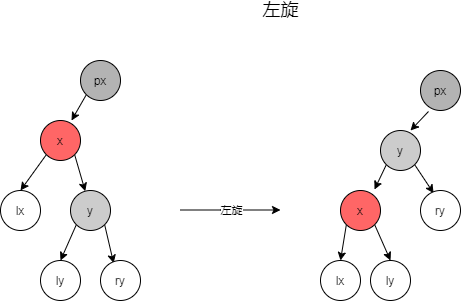java 实现如下：

/**
* 对红黑树的节点(x)进行左旋转
* @param x 节点
* @since 0.0.5
*/
private void leftRotate(Node<T> x) {
// 设置x的右孩子为y
Node<T> y = x.right;
// 将 “y的左孩子” 设为 “x的右孩子”；
// 如果y的左孩子非空，将 “x” 设为 “y的左孩子的父亲”
x.right = y.left;
if (y.left != null)
y.left.parent = x;
// 将 “x的父亲” 设为 “y的父亲”
y.parent = x.parent;
if (x.parent == null) {
this.root = y;            // 如果 “x的父亲” 是空节点，则将y设为根节点
} else {
if (x.parent.left == x)
x.parent.left = y;    // 如果 x是它父节点的左孩子，则将y设为“x的父节点的左孩子”
else
x.parent.right = y;    // 如果 x是它父节点的左孩子，则将y设为“x的父节点的左孩子”
}
// 将 “x” 设为 “y的左孩子”
y.left = x;
// 将 “x的父节点” 设为 “y”
x.parent = y;
}


### 右旋转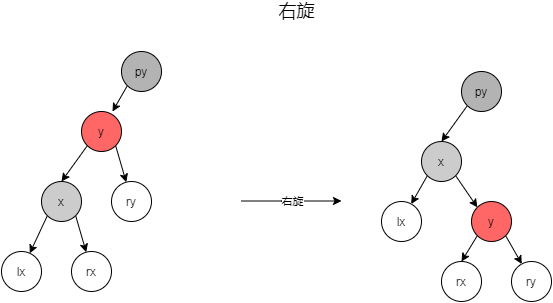java 实现如下：

/**
* 对红黑树的节点(y)进行右旋转
* @param y 节点
* @since 0.0.5
*/
private void rightRotate(Node<T> y) {
// 设置x是当前节点的左孩子。
Node<T> x = y.left;
// 将 “x的右孩子” 设为 “y的左孩子”；
// 如果"x的右孩子"不为空的话，将 “y” 设为 “x的右孩子的父亲”
y.left = x.right;
if (x.right != null)
x.right.parent = y;
// 将 “y的父亲” 设为 “x的父亲”
x.parent = y.parent;
if (y.parent == null) {
this.root = x;            // 如果 “y的父亲” 是空节点，则将x设为根节点
} else {
if (y == y.parent.right)
y.parent.right = x;    // 如果 y是它父节点的右孩子，则将x设为“y的父节点的右孩子”
else
y.parent.left = x;    // (y是它父节点的左孩子) 将x设为“x的父节点的左孩子”
}
// 将 “y” 设为 “x的右孩子”
x.right = y;
// 将 “y的父节点” 设为 “x”
y.parent = x;
}


### 变色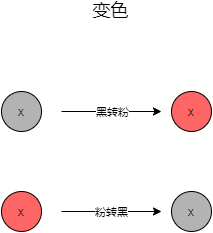java 实现如下：

/**
* 变色-直接取反即可
* @param color 颜色
* @return 变色后的颜色
* @since 0.0.5
*/
private boolean changeColor(boolean color) {
return !color;
}


## 添加

(1) 每个节点或者是黑色，或者是红色。

(2) 根节点是黑色。

(3) 每个叶子节点是黑色。 (注意：这里叶子节点，是指为空的叶子节点)

(4) 如果一个节点是红色的，则它的子节点必须是黑色的。

(5) 从一个节点到该节点的子孙节点的所有路径上包含相同数目的黑节点。

### java 实现

2 设置元素为红色。

3 执行修正。

/**
* 将结点插入到红黑树中
* @param node 节点
* @since 0.0.5
*/
int cmp;
Node<T> y = null;
Node<T> x = this.root;
// 1.1 将红黑树当作一颗二叉查找树，将节点添加到二叉查找树中。
while (x != null) {
y = x;
cmp = node.data.compareTo(x.data);
if (cmp < 0)
x = x.left;
else
x = x.right;
}
// 1.2 找到合适的位置之后，插入新的节点
node.parent = y;
if (y != null) {
cmp = node.data.compareTo(y.data);
if (cmp < 0)
y.left = node;
else
y.right = node;
} else {
this.root = node;
}

// 2. 设置节点的颜色为红色
node.color = RED;

// 3. 将它重新修正为一颗二叉查找树
}


## 新增修正场景

（1）情况说明：被插入的节点是根节点。

（2）情况说明：被插入的节点的父节点是黑色。

（3）情况说明：被插入的节点的父节点是红色。

### 场景1：叔叔是红色

• 现象说明

• 处理策略

(01) 将“父节点”设为黑色。

(02) 将“叔叔节点”设为黑色。

(03) 将“祖父节点”设为“红色”。

(04) 将“祖父节点”设为“当前节点”(红色节点)；即，之后继续对“当前节点”进行操作。

“当前节点”和“父节点”都是红色，违背“特性(4)”。所以，将“父节点”设置“黑色”以解决这个问题。

• 示意图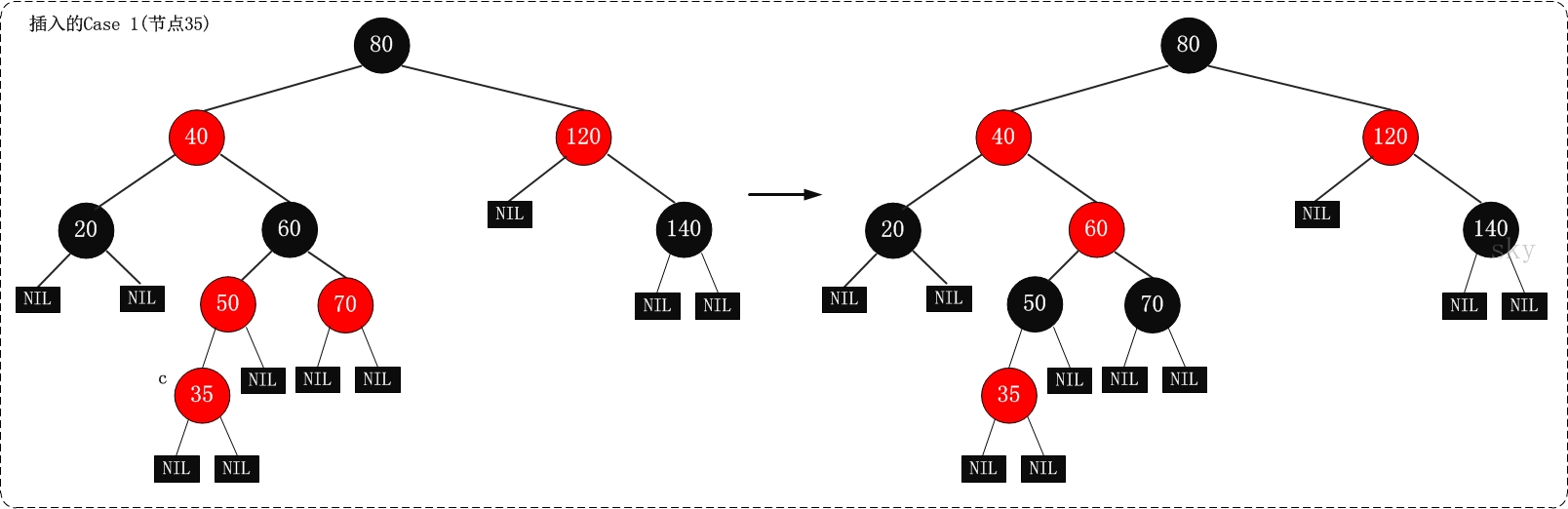### 场景2：叔叔是黑色，且当前节点是右孩子

• 现象说明

• 处理策略

(01) 将“父节点”作为“新的当前节点”。

(02) 以“新的当前节点”为支点进行左旋。

• 示意图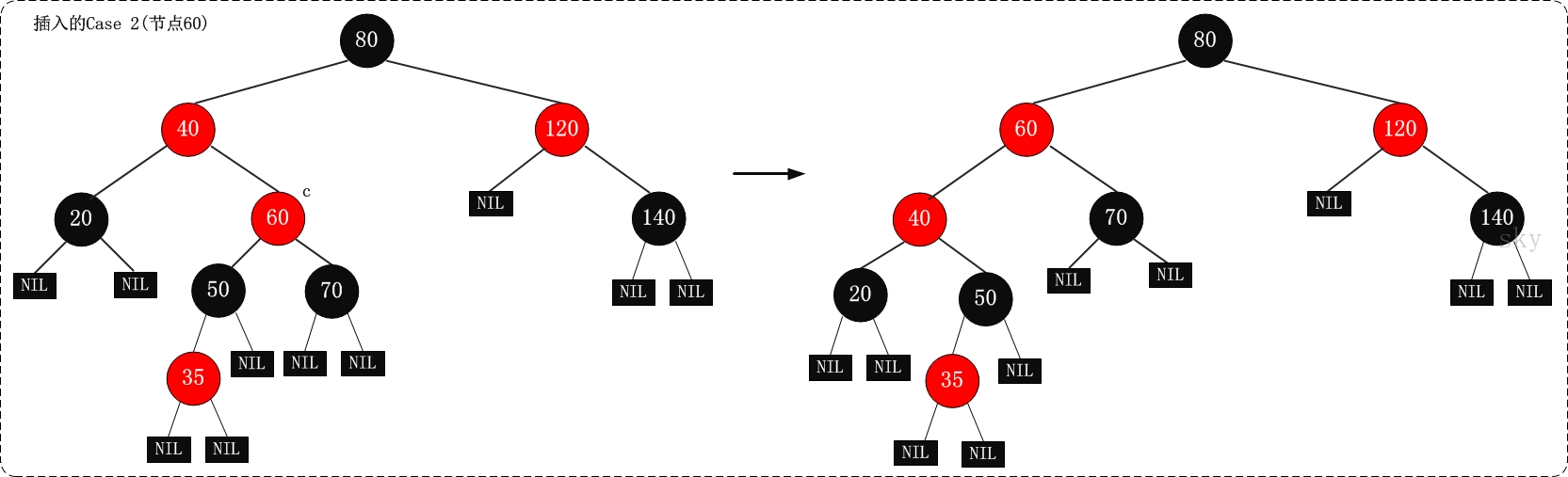### 场景3：叔叔是黑色，且当前节点是左孩子

• 现象说明

• 处理策略

(01) 将“父节点”设为“黑色”。

(02) 将“祖父节点”设为“红色”。

(03) 以“祖父节点”为支点进行右旋。

S和F都是红色，违背了红黑树的“特性(4)”，我们可以将F由“红色”变为“黑色”，就解决了“违背‘特性(4)’”的问题；但却引起了其它问题：违背特性(5)，因为将F由红色改为黑色之后，所有经过F的分支的黑色节点的个数增加了1。

• 示意图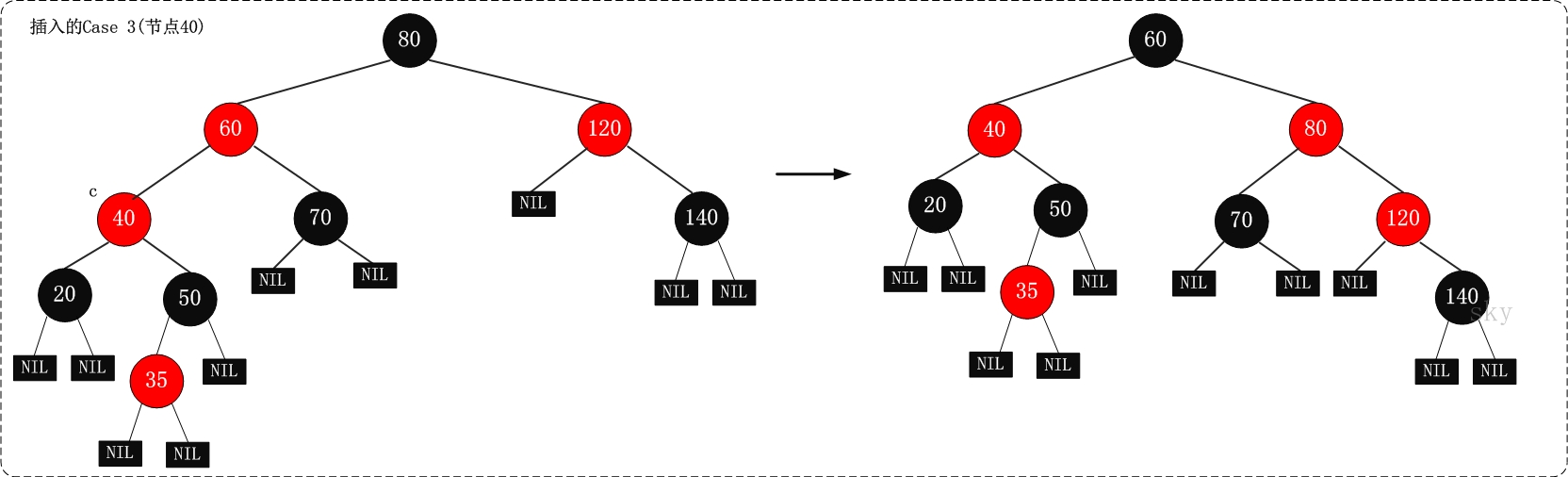## 新增修正实现

java 实现如下：

/**
* 红黑树插入修正函数
*
* 在向红黑树中插入节点之后(失去平衡)，再调用该函数；目的是将它重新塑造成一颗红黑树。
* @param node 插入节点
* @since 0.0.5
*/
Node<T> parent, gparent;
// 若“父节点存在，并且父节点的颜色是红色”
while (((parent = parentOf(node))!=null) && isRed(parent)) {
gparent = parentOf(parent);
//若“父节点”是“祖父节点的左孩子”
if (parent == gparent.left) {
// Case 1条件：叔叔节点是红色
Node<T> uncle = gparent.right;
if ((uncle!=null) && isRed(uncle)) {
setBlack(uncle);
setBlack(parent);
setRed(gparent);
node = gparent;
continue;
}
// Case 2条件：叔叔是黑色，且当前节点是右孩子
if (parent.right == node) {
Node<T> tmp;
leftRotate(parent);
tmp = parent;
parent = node;
node = tmp;
}
// Case 3条件：叔叔是黑色，且当前节点是左孩子。
setBlack(parent);
setRed(gparent);
rightRotate(gparent);
} else {    //若“z的父节点”是“z的祖父节点的右孩子”
// Case 1条件：叔叔节点是红色
Node<T> uncle = gparent.left;
if ((uncle!=null) && isRed(uncle)) {
setBlack(uncle);
setBlack(parent);
setRed(gparent);
node = gparent;
continue;
}
// Case 2条件：叔叔是黑色，且当前节点是左孩子
if (parent.left == node) {
Node<T> tmp;
rightRotate(parent);
tmp = parent;
parent = node;
node = tmp;
}
// Case 3条件：叔叔是黑色，且当前节点是右孩子。
setBlack(parent);
setRed(gparent);
leftRotate(gparent);
}
}
// 将根节点设为黑色
setBlack(this.root);
}


private Node<T> parentOf(Node<T> node) {
return node!=null ? node.parent : null;
}
private boolean colorOf(Node<T> node) {
return node!=null ? node.color : BLACK;
}
private boolean isRed(Node<T> node) {
return (node != null) && (node.color == RED);
}
private boolean isBlack(Node<T> node) {
return !isRed(node);
}
private void setBlack(Node<T> node) {
if (node!=null)
node.color = BLACK;
}
private void setRed(Node<T> node) {
if (node!=null)
node.color = RED;
}
private void setParent(Node<T> node, Node<T> parent) {
if (node!=null)
node.parent = parent;
}
private void setColor(Node<T> node, boolean color) {
if (node!=null)
node.color = color;
}


## 删除元素

（1）被删除节点没有儿子，即为叶节点。那么，直接将该节点删除就OK了。

（2）被删除节点只有一个儿子。那么，直接删除该节点，并用该节点的唯一子节点顶替它的位置。

（3）被删除节点有两个儿子。那么，先找出它的后继节点；然后把“它的后继节点的内容”复制给“该节点的内容”；之后，删除“它的后继节点”。在这里，后继节点相当于替身，在将后继节点的内容复制给”被删除节点”之后，再将后继节点删除。这样就巧妙的将问题转换为”删除后继节点”的情况了，下面就考虑后继节点。 在”被删除节点”有两个非空子节点的情况下，它的后继节点不可能是双子非空。既然”的后继节点”不可能双子都非空，就意味着”该节点的后继节点”要么没有儿子，要么只有一个儿子。若没有儿子，则按”情况（1）”进行处理；若只有一个儿子，则按”情况（2）”进行处理。

### java 实现

@Override
public boolean remove(T data) {
Node<T> node = search(root, data);
if(node == null) {
return false;
}

remove(node);
return true;
}


/**
* (递归实现)查找"红黑树x"中键值为key的节点
* @param x 节点
* @param key 数据
* @return 结果
* @since 0.0.5
*/
private Node<T> search(Node<T> x, T key) {
if (x==null)
return x;
int cmp = key.compareTo(x.data);
if (cmp < 0)
return search(x.left, key);
else if (cmp > 0)
return search(x.right, key);
else
return x;
}


/**
* 删除结点(node)
* @param node 节点
* @since 0.0.5
*/
private void remove(Node<T> node) {
Node<T> child, parent;
boolean color;
// 被删除节点的"左右孩子都不为空"的情况。
if ( (node.left!=null) && (node.right!=null) ) {
// 被删节点的后继节点。(称为"取代节点")
// 用它来取代"被删节点"的位置，然后再将"被删节点"去掉。
Node<T> replace = node;
// 获取后继节点
replace = replace.right;
while (replace.left != null)
replace = replace.left;
// "node节点"不是根节点(只有根节点不存在父节点)
if (parentOf(node)!=null) {
if (parentOf(node).left == node)
parentOf(node).left = replace;
else
parentOf(node).right = replace;
} else {
// "node节点"是根节点，更新根节点。
this.root = replace;
}
// child是"取代节点"的右孩子，也是需要"调整的节点"。
// "取代节点"肯定不存在左孩子！因为它是一个后继节点。
child = replace.right;
parent = parentOf(replace);
// 保存"取代节点"的颜色
color = colorOf(replace);
// "被删除节点"是"它的后继节点的父节点"
if (parent == node) {
parent = replace;
} else {
// child不为空
if (child!=null)
setParent(child, parent);
parent.left = child;
replace.right = node.right;
setParent(node.right, replace);
}
replace.parent = node.parent;
replace.color = node.color;
replace.left = node.left;
node.left.parent = replace;
if (color == BLACK)
removeFixUp(child, parent);
node = null;
return ;
}
if (node.left !=null) {
child = node.left;
} else {
child = node.right;
}
parent = node.parent;
// 保存"取代节点"的颜色
color = node.color;
if (child!=null)
child.parent = parent;
// "node节点"不是根节点
if (parent!=null) {
if (parent.left == node)
parent.left = child;
else
parent.right = child;
} else {
this.root = child;
}
if (color == BLACK)
removeFixUp(child, parent);
node = null;
}


## 删除修正

(1) 每个节点或者是黑色，或者是红色。

(2) 根节点是黑色。

(3) 每个叶子节点是黑色。(注意：这里叶子节点，是指为空的叶子节点)

(4) 如果一个节点是红色的，则它的子节点必须是黑色的。

(5) 从一个节点到该节点的子孙节点的所有路径上包含相同数目的黑节点。

a) x指向一个”红+黑”节点。此时，将x设为一个”黑”节点即可。

b) x指向根。此时，将x设为一个”黑”节点即可。

c) 非前面两种姿态。

（1）情况说明：x是“红+黑”节点。

（2）情况说明：x是“黑+黑”节点，且x是根。

（3）情况说明：x是“黑+黑”节点，且x不是根。

### 场景1：x是”黑+黑”节点，x的兄弟节点是红色

• 现象说明

x是”黑+黑”节点，x的兄弟节点是红色。(此时x的父节点和x的兄弟节点的子节点都是黑节点)。

• 处理策略

(01) 将x的兄弟节点设为“黑色”。

(02) 将x的父节点设为“红色”。

(03) 对x的父节点进行左旋。

(04) 左旋后，重新设置x的兄弟节点。

• 示意图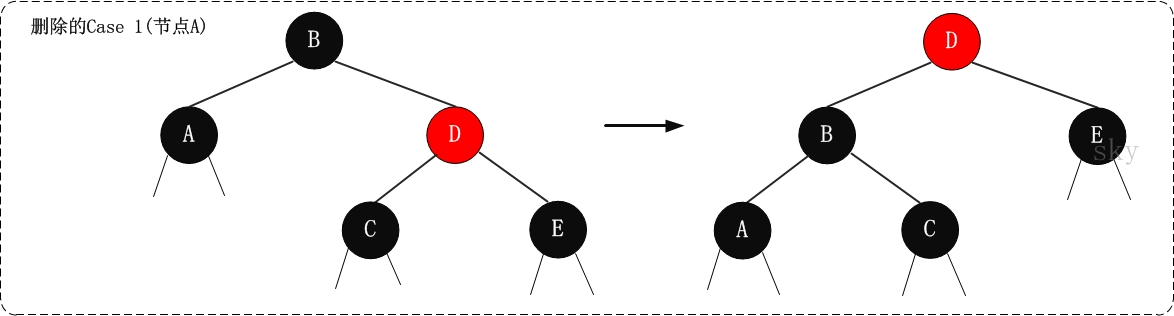### 场景2： x是”黑+黑”节点，x的兄弟节点是黑色，x的兄弟节点的两个孩子都是黑色

• 现象说明

x是“黑+黑”节点，x的兄弟节点是黑色，x的兄弟节点的两个孩子都是黑色。

• 处理策略

(01) 将x的兄弟节点设为“红色”。

(02) 设置“x的父节点”为“新的x节点”。

x是“黑+黑”节点，我们将x由“黑+黑”节点 变成 “黑”节点，多余的一个“黑”属性移到x的父节点中，即x的父节点多出了一个黑属性(若x的父节点原先是“黑”，则此时变成了“黑+黑”；若x的父节点原先时“红”，则此时变成了“红+黑”)。

• 示意图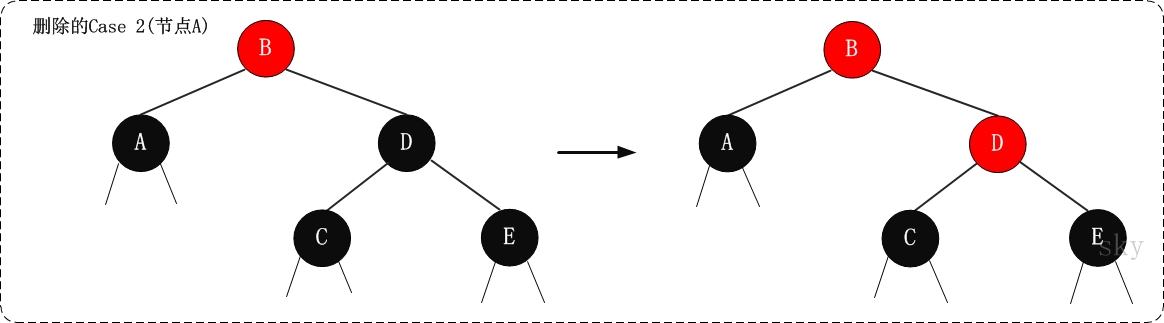### 场景3：x是“黑+黑”节点，x的兄弟节点是黑色；x的兄弟节点的左孩子是红色，右孩子是黑色的

• 现象说明

x是“黑+黑”节点，x的兄弟节点是黑色；x的兄弟节点的左孩子是红色，右孩子是黑色的。

• 处理策略

(01) 将x兄弟节点的左孩子设为“黑色”。

(02) 将x兄弟节点设为“红色”。

(03) 对x的兄弟节点进行右旋。

(04) 右旋后，重新设置x的兄弟节点。

• 示意图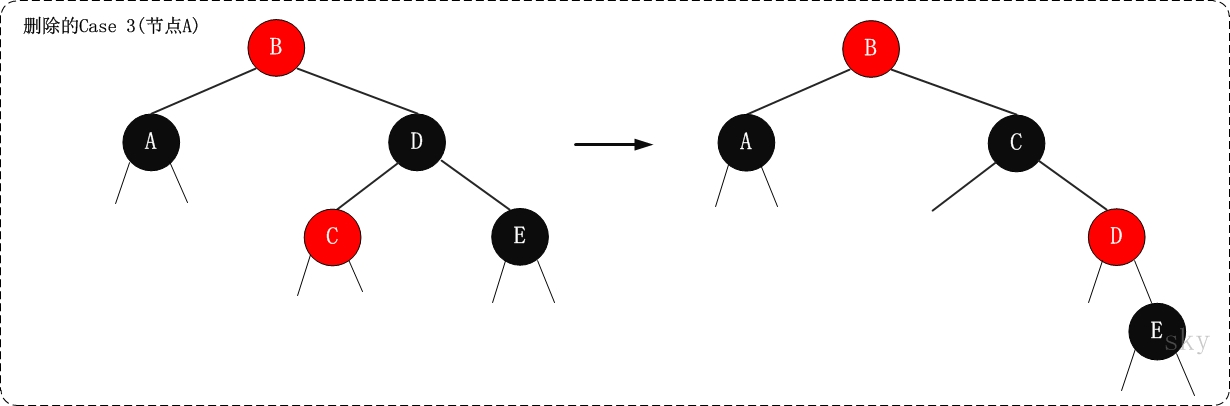### 场景4：x是“黑+黑”节点，x的兄弟节点是黑色；x的兄弟节点的右孩子是红色的，x的兄弟节点的左孩子任意颜色

• 现象说明

x是“黑+黑”节点，x的兄弟节点是黑色；x的兄弟节点的右孩子是红色的，x的兄弟节点的左孩子任意颜色。

• 处理策略

(01) 将x父节点颜色 赋值给 x的兄弟节点。

(02) 将x父节点设为“黑色”。

(03) 将x兄弟节点的右子节设为“黑色”。

(04) 对x的父节点进行左旋。

(05) 设置“x”为“根节点”。

• 为什么？

• 示意图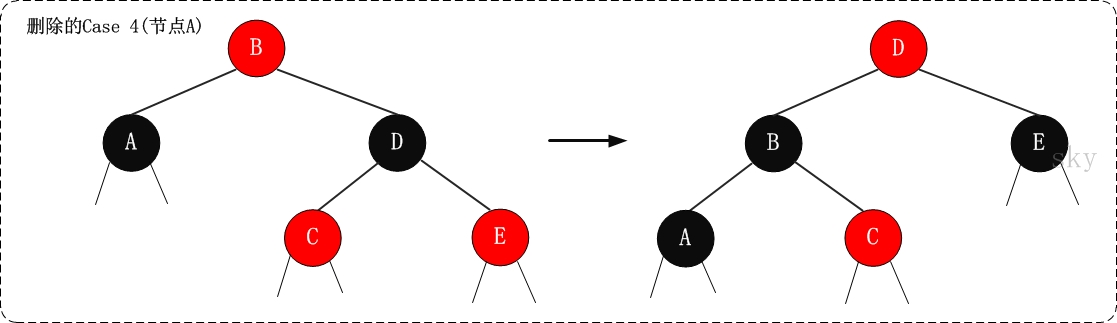### java 实现

/**
* 红黑树删除修正函数
* @param node 节点
* @param parent 父节点
* @since 0.0.5
*/
private void removeFixUp(Node<T> node, Node<T> parent) {
Node<T> other;
while ((node==null || isBlack(node)) && (node != this.root)) {
if (parent.left == node) {
other = parent.right;
if (isRed(other)) {
// Case 1: x的兄弟w是红色的
setBlack(other);
setRed(parent);
leftRotate(parent);
other = parent.right;
}
if ((other.left==null || isBlack(other.left)) &&
(other.right==null || isBlack(other.right))) {
// Case 2: x的兄弟w是黑色，且w的俩个孩子也都是黑色的
setRed(other);
node = parent;
parent = parentOf(node);
} else {
if (other.right==null || isBlack(other.right)) {
// Case 3: x的兄弟w是黑色的，并且w的左孩子是红色，右孩子为黑色。
setBlack(other.left);
setRed(other);
rightRotate(other);
other = parent.right;
}
// Case 4: x的兄弟w是黑色的；并且w的右孩子是红色的，左孩子任意颜色。
setColor(other, colorOf(parent));
setBlack(parent);
setBlack(other.right);
leftRotate(parent);
node = this.root;
break;
}
} else {
other = parent.left;
if (isRed(other)) {
// Case 1: x的兄弟w是红色的
setBlack(other);
setRed(parent);
rightRotate(parent);
other = parent.left;
}
if ((other.left==null || isBlack(other.left)) &&
(other.right==null || isBlack(other.right))) {
// Case 2: x的兄弟w是黑色，且w的俩个孩子也都是黑色的
setRed(other);
node = parent;
parent = parentOf(node);
} else {
if (other.left==null || isBlack(other.left)) {
// Case 3: x的兄弟w是黑色的，并且w的左孩子是红色，右孩子为黑色。
setBlack(other.right);
setRed(other);
leftRotate(other);
other = parent.left;
}
// Case 4: x的兄弟w是黑色的；并且w的右孩子是红色的，左孩子任意颜色。
setColor(other, colorOf(parent));
setBlack(parent);
setBlack(other.left);
rightRotate(parent);
node = this.root;
break;
}
}
}
if (node!=null)
setBlack(node);
}


# 测试代码

## 测试

public class RedBlackTreeTest {

private static final int a[] = {10, 40, 30, 60, 90, 70, 20, 50, 80};

@Test
public void helloTest() {
ISortTree<Integer> tree = new RedBlackTree<>();

for (int i = 0; i < a.length; i++) {
}

System.out.println("树的详细信息");
tree.print();

// 删除
tree.remove(10);
System.out.println("删除 10 之后的详细信息：");
tree.print();
}

}


### 日志

树的详细信息
30(B) is root
10(B) is 30's   left child
20(R) is 10's  right child
60(R) is 30's  right child
40(B) is 60's   left child
50(R) is 40's  right child
80(B) is 60's  right child
70(R) is 80's   left child
90(R) is 80's  right child

30(B) is root
20(B) is 30's   left child
60(R) is 30's  right child
40(B) is 60's   left child
50(R) is 40's  right child
80(B) is 60's  right child
70(R) is 80's   left child
90(R) is 80's  right child


JAVA学习-红黑树详解